# Viridisify¶

As usual this is available and as been written as a jupyter notebook if you like to play with the code feel free to fork it.

The jet colormap (AKA "rainbow") is ubiquitous, there are a lot of controverse as to wether it is (from far) the best one. And better options have been designed.

The question is, if you have a graph that use a specific colormap, and you would prefer for it to use another one; what do you do ?

Well is you have th eunderlying data that's easy, but it's not always the case.

So how to remap a plot which has a non perceptually uniform colormap using another ? What's happend if yhere are encoding artificats and my pixels colors are slightly off ?

I came up with a prototype a few month ago, and was asked recently by @stefanv to "correct" a animated plot of huricane Matthew, where the "jet" colormap seem to provide an illusion of growth:

Let's see how we can convert a "Jet" image to a viridis based one. We'll first need some assumptions:

• This assume that you "know" the initial color map of a plot, and that the emcoding/compressing process of the plot will not change the colors "too much".
• There are pixels in the image which are not part of the colormap (typically text, axex, cat pictures....)

We will try to remap all the pixels that fall not "too far" from the initial colormap to the new colormap.

In :
%matplotlib inline
import matplotlib
import matplotlib.pyplot as plt
import numpy as np

In :
import matplotlib.colors as colors

In :
!rm *.png *.gif out*

rm: output.gif: No such file or directory


I used the following to convert from mp4 to image sequence (8 fps determined manually). Sequence of images to video, and video to gif (quality is better than to gif dirrectly):

$ffmpeg -i INPUT.mp4 -r 8 -f image2 img%02d.png$ ffmpeg -framerate 8 -i vir-img%02d.png -c:v libx264 -r 8 -pix_fmt yuv420p out.mp4
\$ ffmpeg -i out.mp4  output.gif
In :
%%bash
ffmpeg -i input.mp4 -r 8 -f image2 img%02d.png -loglevel panic


Let's take our image without the alpha channel, so only the first 3 components:

In :
import matplotlib.image as mpimg

In :
fig, ax = plt.subplots()
ax.imshow(img)
fig.set_figheight(10)
fig.set_figwidth(10)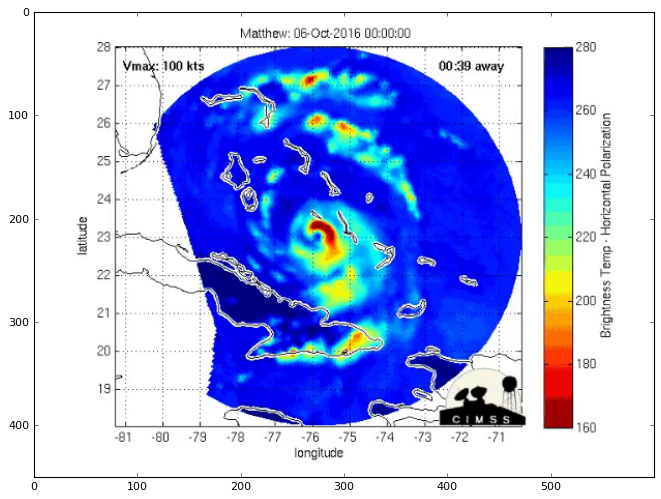As you can see it does use "Jet" (most likely),

let's look at the repartitions of pixels on the RGB space...

In :
import numpy as np
from mpl_toolkits.mplot3d import Axes3D

import matplotlib.pyplot as plt

In :
def rep(im, cin=None, sub=128):
fig = plt.figure()
pp = im.reshape((-1,3)).T[:,::300]

if cin:
cmapin = plt.get_cmap(cin)
cmap256 = colors.makeMappingArray(sub, cmapin)[:, :3].T
ax.scatter(cmap256, cmap256, cmap256, marker='.', label='colormap', c=range(sub), cmap=cin, edgecolor=None)

ax.scatter(pp, pp, pp, c=pp.T, marker='+')

ax.set_xlabel('R')
ax.set_ylabel('G')
ax.set_zlabel('B')
ax.set_title('Color of pixels')
if cin:
ax.legend()
return ax

ax = rep(img)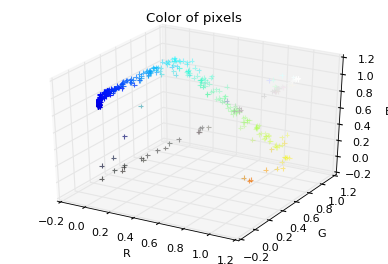We can see a specific clusers of pixel, let's plot the location of our "Jet" colormap and a diagonal of "gray". We can guess the effect of various compressions artifacts have jittered the pixels slightly away from their original location.

Let's look at where the jet colormap is supposed to fall:

In :
rep(img, 'jet')

Out:
<matplotlib.axes._subplots.Axes3DSubplot at 0x111c9cc88>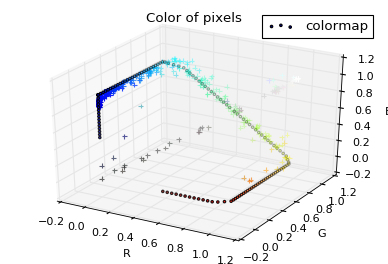Ok, that's pretty accurate, we also see that our selected graph does nto use the full extent of jet.

in order to find all the pixels that uses "Jet" efficiently we will use scipy.spatial.KDTree in the colorspace. In particular we will subsample the initial colormap in sub=256 subsamples, and collect only pixels that are within d=0.2 of this subsample, and map each of these pixels to the closer subsample.

As we know the subsampling of the initial colormap, we can also determine the output colors.

The Pixels that are "too far" from the pixels of the colormap are keep unchanged.

increasing 256 to higher value will give a smoother final colormap.

In :
from scipy.spatial import cKDTree

In :
def convert(sub=256, d=0.2, cin='jet', cout='viridis', img=img, show=True):
viridis = plt.get_cmap(cout)
cmapin = plt.get_cmap(cin)
cmap256 = colors.makeMappingArray(sub, cmapin)[:, :3]
original_shape = img.shape
img_data = img.reshape((-1,3))

# this will efficiently find the pixels "close" to jet
# and assign them to which point (from 1 to 256) they are on the colormap.
K = cKDTree(cmap256)
res = K.query(img_data, distance_upper_bound=d)

indices = res
l = len(cmap256)
indices = indices.reshape(original_shape[:2])
remapped = indices

indices.max()

remapped = remapped / (l-1)

# here we add only these pixel and plot them again with viridis.
if show:
fig, ax = plt.subplots()
fig.set_figheight(10)
fig.set_figwidth(10)
ax.imshow(blend)
return blend

In :
res = convert(img=img)
rep(res)

Out:
<matplotlib.axes._subplots.Axes3DSubplot at 0x113791278>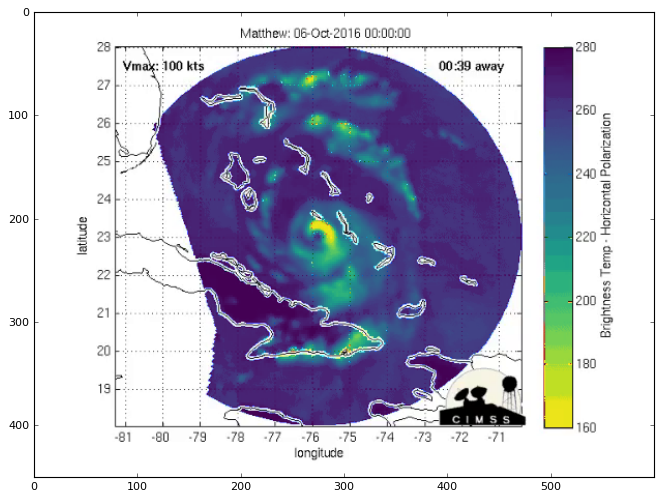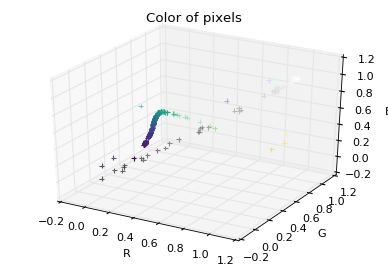Let's loot at what happend if we decrease our leniency for the "proximity" of each pixel to the jet colormap:

In :
rep(convert(img=img, d=0.05))

Out:
<matplotlib.axes._subplots.Axes3DSubplot at 0x1159fd6d8>